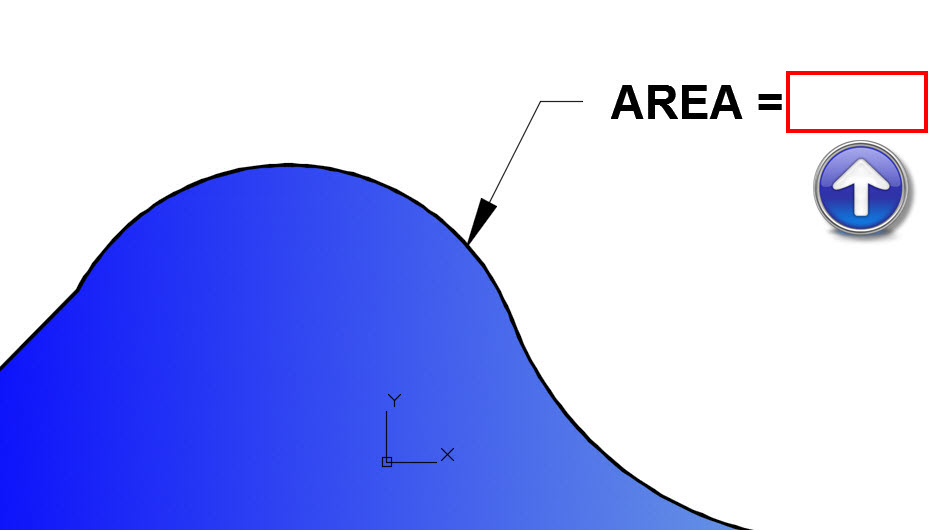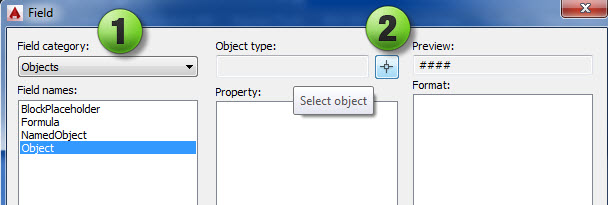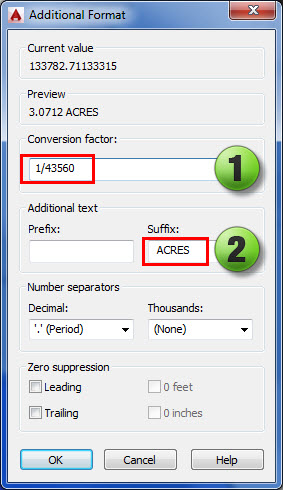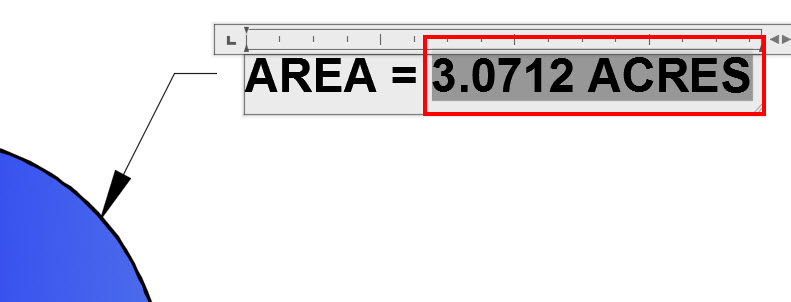I am sure we have all had to calculate an area of a pond, footprint, or some other irregular shape in AutoCAD. What about those times when we need to convert to acres, simple – right? We just get out our calculator or use the onscreen calculator and do the math. Let’s let AutoCAD do that for us. We are now going to locate or create a closed object or polyline in a drawing and place a leader with mtext on an object and use a field to label the area (in acres) of the object. Let’s first drawing an irregular shape on our drawing. Next type the mleader command and place a leader pointing to the object as shown in Figure 1

Figure 1: Object – Mtext – LabelNotice how we do not have anything after the “=” sign for the area. Right click and highlight (red box) where you want the number to be in your mtext and select insert field as shown in Figure 2.

Figure 2: Right click-Insert FieldYou now need to change the field category to Objects (1) and then hit the Object type button (2) and you will be sent back to out to the AutoCAD screen to select the object.

Figure 3: Select the ObjectSelect the outer bold line (boundary) in your drawing. The field selection window will pop up. Select Area then Decimal. But wait, we want acres and this is in standard units 1-1 which would be square feet. We are assuming that the coordinate system is setup to decimal units or 1-1. Therefore the value displayed in the field will be in square feet and not acres. We just need to additional format to the field. Checking the coordinate system is very important for any type of calculation. Make sure you are in the correct coordinate system and your drawing units are correct.

Figure 4: Field selectionsSelect the additional format button as shown above in Figure 4 above and  another dialog box will pop up and you can add some custom formatting.  We need to convert the value to Acres and we also would like to add a suffix as shown in Figure 5 .

• Step 1 you are going to divide the value by 43,560 which is how many square feet are in an acre.
• Step 2 you will add a Suffix, in this case we are adding the word ACRES.You now have linked a field with additional format to an object in AutoCAD. If you revise the boundary the field will automatically update with the new area shown in Acres. If you break the link between the object and the field, simply right click and reselect the object following the steps above. Your final output should look just like Figure 6 below. Don’t forget to change the precision to your desired output, you can change this in the additional format window as shown in Figure 4.

Figure 6: Area Calculation Shown1.Yes, if you link the field to that object the value will automatically change. Do a regen after you change the size and notice the number will change.

2.That’s helps. Thanks. But if i change the size of that object, will area will change automatically or i have to redo the process?

3.You can use a mathematical expression in the field formula dialog box – then if the areas changed the sum would change. Great question I will test it out.

4.5.6.